Introduction¶

This notebook is an implementation of Jón Daníelsson's Financial Risk Forecasting (Wiley, 2011) in MATLAB 2019b, with annotations and introductory examples. The introductory examples (Appendix) are similar to Appendix C in the original book.

The following code has been adapted from the original, implemented in MATLAB 2019b. Occasional lines of code which require different syntax to run in MATLAB 2019b are also noted in trailing comments.

Bullet point numbers correspond to the MATLAB Listing numbers in the original book, referred to henceforth as FRF.

More details can be found at the book website: https://www.financialriskforecasting.com/

Last updated: July 2020

Copyright 2011-2020 Jón Daníelsson. This code is free software: you can redistribute it and/or modify it under the terms of the GNU General Public License as published by the Free Software Foundation, either version 3 of the License, or (at your option) any later version. This code is distributed in the hope that it will be useful, but WITHOUT ANY WARRANTY; without even the implied warranty of MERCHANTABILITY or FITNESS FOR A PARTICULAR PURPOSE. See the GNU General Public License for more details. The GNU General Public License is available at: https://www.gnu.org/licenses/.

Appendix: An Introduction to MATLAB¶

Created in MATLAB 2019b (July 2020)

• M.1: Entering and Printing Data
• M.2: Vectors, Matrices and Sequences
• M.3: Importing Data (to be updated)
• M.4: Basic Summary Statistics
• M.5: Calculating Moments
• M.6: Basic Matrix Operations
• M.7: Statistical Distributions
• M.8: Statistical Tests
• M.9: Time Series
• M.10: Loops and Functions
• M.11: Basic Graphs
• M.12: Miscellaneous Useful Functions
In :
% Entering and Printing Data
% Listing M.1
% Last updated June 2018
%
%

x = 10;             % assign x the value 10, silencing output print with ;
disp(x)             % display x
10

In :
% Vectors, Matrices and Sequences
% Listing M.2
% Last updated June 2018
%
%

y = [1,3,5,7,9]            % lists are denoted by square brackets

y(3)                       % calling 3rd element (MATLAB indices start at 1)

size(y)                    % shows that y is 1 x 5 (a row vector, by default)

length(y)                  % as expected, y has length 5

v = nan(2,3)               % fill a 2 x 3 matrix with NaN values

size(v)                    % as expected, v is size (2,3)

w = repmat([1,2,3]', 2, 3) % repeats matrix twice by rows, thrice by columns

s = 1:10                   % s is a list of integers from 1 to 10 inclusive
y =

1     3     5     7     9

ans =

5

ans =

1     5

ans =

5

v =

NaN   NaN   NaN
NaN   NaN   NaN

ans =

2     3

w =

1     1     1
2     2     2
3     3     3
1     1     1
2     2     2
3     3     3

s =

1     2     3     4     5     6     7     8     9    10

In :
% Basic Summary Statistics
% Listing M.3
% Last updated July 2020
%
%

y = [3.14; 15; 9.26; 5];    % List with semicolons is a column vector

sum(y)         % sum of all elements of y
prod(y)        % product of all elements of y
max(y)         % maximum value of y
min(y)         % minimum value of y
range(y)       % min, max value of y
mean(y)        % arithmetic mean
median(y)      % median
var(y)         % variance
cov(y)         % covar matrix = variance for single vector
corrcoef(y)    % corr matrix =  for single vector
sort(y)        % sorting in ascending order
log(y)         % natural log
ans =

32.4000

ans =

2.1807e+03

ans =

15

ans =

3.1400

ans =

11.8600

ans =

8.1000

ans =

7.1300

ans =

27.7224

ans =

27.7224

ans =

1

ans =

3.1400
5.0000
9.2600
15.0000

ans =

1.1442
2.7081
2.2257
1.6094

In :
% Calculating Moments
% Listing M.4
% Last updated June 2018
%
%

mean(y)      % mean
var(y)       % variance
std(y)       % unbiased standard deviation, by default
skewness(y)  % skewness
kurtosis(y)  % kurtosis
ans =

8.1000

ans =

27.7224

ans =

5.2652

ans =

0.4700

ans =

1.7153

In :
% Basic Matrix Operations
% Listing M.5
% Last updated June 2018
%
%

z = [1, 2; 3, 4]  % z is a 2 x 2 matrix (Note the use of ; as row separator)
x = [1, 2]        % x is a 1 x 2 matrix

%% Note: z * x is undefined since the two matrices are not conformable

z * x'            % this evaluates to a 2 x 1 matrix

vertcat(z,x)      % "stacking" z and x vertically
horzcat(z,x')     % "stacking z and x' horizontally

%% Note: dimensions must match along the combining axis)
z =

1     2
3     4

x =

1     2

ans =

5
11

ans =

1     2
3     4
1     2

ans =

1     2     1
3     4     2

In :
% Statistical Distributions
% Listing M.6
% Last updated June 2018
%
%

q = -3:1:3                       % specify a set of values

p = 0.1:0.1:0.9                  % specify a set of probabilities

norminv(p, 0, 1)                 % element-wise inverse Normal quantile

tcdf(q, 4)                       % element-wise cdf under Student-t(4)

chi2pdf(q, 2)                    % element-wise pdf under Chisq(2)

%% One can also obtain pseudorandom samples from distributions

x = trnd(5, 100, 1);             % Sampling 100 times from t dist with 5 df

y = normrnd(0, 1, 100, 1);       % Sampling 50 times from a standard normal

%% Given sample data, we can also obtain MLE estimates of distribution parameters:

res = fitdist(x, "Normal")       % Fitting x to normal dist
q =

-3    -2    -1     0     1     2     3

p =

Columns 1 through 7

0.1000    0.2000    0.3000    0.4000    0.5000    0.6000    0.7000

Columns 8 through 9

0.8000    0.9000

ans =

Columns 1 through 7

-1.2816   -0.8416   -0.5244   -0.2533         0    0.2533    0.5244

Columns 8 through 9

0.8416    1.2816

ans =

0.0200    0.0581    0.1870    0.5000    0.8130    0.9419    0.9800

ans =

0         0         0    0.5000    0.3033    0.1839    0.1116

res =

NormalDistribution

Normal distribution
mu = 0.146605   [-0.155625, 0.448836]
sigma =  1.52317   [1.33736, 1.76943]

In :
% Statistical Tests
% Listing M.7
% Last updated July 2020
%
%

x = trnd(5, 500, 1);                    % Create hypothetical dataset x

[h1, p1, jbstat] = jbtest(x)            % Jarque-Bera test for normality

[h2, p2, lbstat] = lbqtest(x,'lags',20) % Ljung-Box test for serial correlation - Needs Econometrics Toolbox
Warning: P is less than the smallest tabulated value, returning 0.001.
> In jbtest (line 136)

h1 =

1

p1 =

1.0000e-03

jbstat =

398.3104

h2 =

logical

0

p2 =

0.1588

lbstat =

26.2154

In :
% Time Series
% Listing M.8
% Last updated June 2018
%
%

x = trnd(5, 60, 1); % Create hypothetical dataset x

subplot(1,2,1)
autocorr(x, 20)     % autocorrelation for lags 1:20
subplot(1,2,2)
parcorr(x,20)       % partial autocorrelation for lags 1:20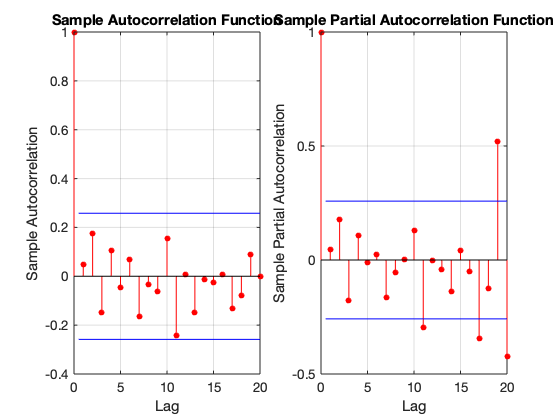In :
% Loops and Functions
% Listing M.9
% Last updated June 2018
%
%

%% For loops

for i = 3:7        % iterates through [3,4,5,6,7]
i^2
end

%% If-else loops

X = 10;

if (rem(X,3) == 0)
disp("X is a multiple of 3")
else
disp("X is not a multiple of 3")
end

%% Functions (example: a simple excess kurtosis function)
%% NOTE: in MATLAB, functions can be defined in 2 locations:
%% 1) in a separate file (e.g. excess_kurtosis.m in this case) in the workspace
%% 2) in the same file as the rest of the code, BUT at the end of the file

%% function k = excess_kurtosis(x, excess)
%%     if nargin == 1                      % if there is only 1 argument
%%         excess = 3;                     % set excess = 3
%%     end                                 % this is how optional param excess is set
%%     m4 = mean((x-mean(x)).^4);
%%     k = m4/(std(x)^4) - excess;
%% end
ans =

9

ans =

16

ans =

25

ans =

36

ans =

49

X is not a multiple of 3
In :
% Basic Graphs
% Listing M.10
% Last updated July 2020
%
%

y = normrnd(0, 1, 50, 1);
z = trnd(4, 50, 1);

subplot(2,2,1)
bar(y)            % bar plot
title("Bar plot")
subplot(2,2,2)
plot(y)           % line plot
title("Line plot")
subplot(2,2,3)
histogram(y)      % histogram
title("Histogram")
subplot(2,2,4)
scatter(y,z)      % scatter plot
title("Scatter plot")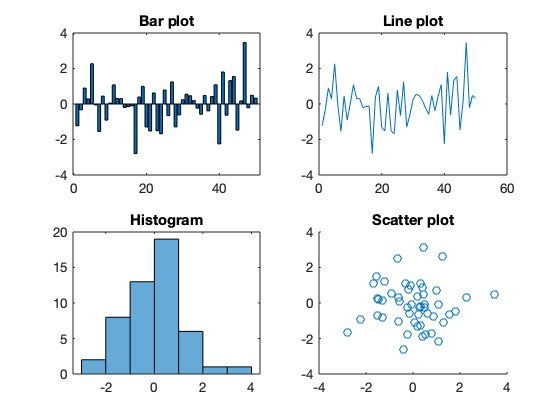In :
% Miscellaneous Useful Functions
% Listing M.11
% Last updated June 2018
%
%

%% Convert objects from one type to another with int8() etc
%% To check type, use isfloat(object), isinteger(object) and so on
x = 8.0;
isfloat(x)
x = int8(x);
isinteger(x)
ans =

logical

1

ans =

logical

1

Chapter 1: Financial Markets, Prices and Risk¶

• 1.3/1.4: Summary statistics for returns timeseries
• 1.5/1.6: Autocorrelation function (ACF) plots, Ljung-Box test
• 1.7/1.8: Quantile-Quantile (QQ) plots
• 1.9/1.10: Correlation matrix between different stocks
In :
% Listing 1.2
% Last updated July 2020
%
%

y=diff(log(price)); % calculate returns

plot(y)             % plot returns
title("S&P500 returns")

% END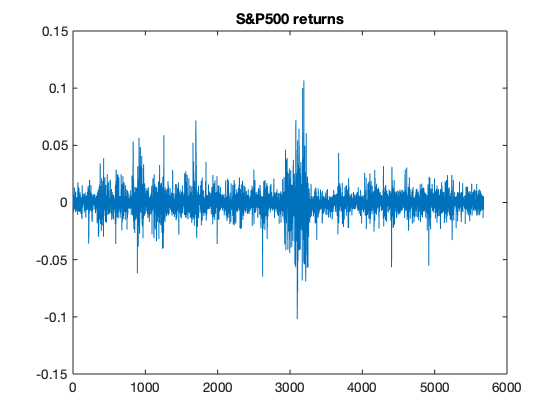In :
% Sample statistics in MATLAB
% Listing 1.4
% Last updated July 2020
%
%

mean(y)
std(y)
min(y)
max(y)
skewness(y)
kurtosis(y)
[h,pValue,stat]=jbtest(y);

%% NOTE: in MATLAB some functions require name-value pairs
%% e.g. [h,pValue,stat]=jbtest(y);
ans =

2.5816e-04

ans =

0.0100

ans =

-0.1020

ans =

0.1067

ans =

0.1526

ans =

16.9812

Warning: P is less than the smallest tabulated value, returning 0.001.
> In jbtest (line 136)
In :
% ACF plots and the Ljung-Box test in MATLAB
% Listing 1.6
% Last updated July 2020
%
%

%% subplots here are just for ease of visualization

subplot(1,2,1)
autocorr(y, 20)
subplot(1,2,2)
autocorr(y.^2, 20)

[h,pValue,stat]=lbqtest(y,'lags',20);
[h,pValue,stat]=lbqtest(y.^2,'lags',20);
h =

logical

1

pValue =

1.8088e-11

stat =

93.4881

h =

logical

1

pValue =

0

stat =

2.5430e+03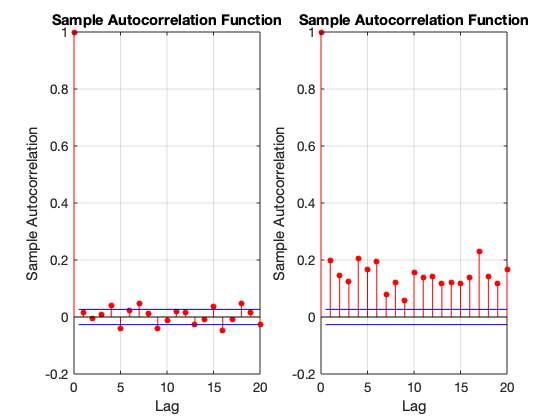In :
% QQ plots in MATLAB
% Listing 1.8
% Last updated 2011
%
%

%% subplots here are just for ease of visualization

subplot(1,2,1)
qqplot(y)
subplot(1,2,2)
qqplot(y, fitdist(y,'tLocationScale'))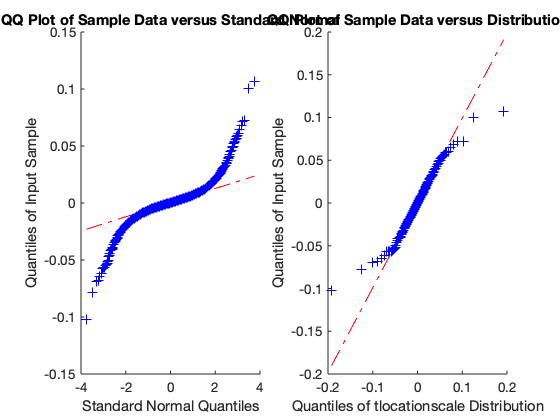In :
% Listing 1.10
% Last updated 2011
%
%

y=diff(log(price));
corr(y) % correlation matrix
ans =

1.0000    0.2297    0.2126
0.2297    1.0000    0.1451
0.2126    0.1451    1.0000

Chapter 2: Univariate Volatility Modelling¶

• 2.1/2.2: GARCH and t-GARCH estimation
• 2.3/2.4: APARCH estimation
In :
?tarch
In :
% ARCH and GARCH estimation in MATLAB
% Listing 2.2
% Last updated June 2018
%
%

y=diff(log(p))*100;
y=y-mean(y);

%% We multiply returns by 100 and de-mean them

tarch(y,1,0,0);              % ARCH(1)
tarch(y,4,0,0);              % ARCH(4)
tarch(y,4,0,1);              % GARCH(4,1)
tarch(y,1,0,1);              % GARCH(1,1)
tarch(y,1,0,1,'STUDENTST');  % t-GARCH(1,1)
____________________________________________________________
Diagnostic Information

Number of variables: 2

Functions
Objective:                            tarch_likelihood
Hessian:                              finite-differencing (or Quasi-Newton)

Algorithm selected
quasi-newton

____________________________________________________________
End diagnostic information
First-order
Iteration  Func-count       f(x)        Step-size       optimality
0           3          7868.18                           974
1           9          7687.07    0.000391318           48.7
2          15          7679.24             10           39.5
3          18          7671.31              1           46.3
4          21          7670.68              1           14.9
5          24          7670.62              1          0.659
6          27          7670.62              1         0.0125
7          30          7670.62              1        0.00317

Local minimum found.

Optimization completed because the size of the gradient is less than
the value of the optimality tolerance.

____________________________________________________________
Diagnostic Information

Number of variables: 5

Functions
Objective:                            tarch_likelihood
Hessian:                              finite-differencing (or Quasi-Newton)

Algorithm selected
quasi-newton

____________________________________________________________
End diagnostic information
First-order
Iteration  Func-count       f(x)        Step-size       optimality
0           6          7496.14                           339
1          18          7457.23    0.000576643           64.7
2          30          7395.22             10           54.5
3          36          7321.82              1           82.4
4          42          7292.66              1             31
5          48          7291.69              1           24.8
6          54          7290.25              1           18.6
7          60          7286.58              1             27
8          66          7282.48              1           39.3
9          72          7280.01              1           27.3
10          78          7279.39              1           9.96
11          84          7279.27              1           4.89
12          90          7279.25              1           4.88
13          96          7279.18              1           5.18
14         102          7279.09              1           7.43
15         108          7278.98              1           6.51
16         114          7278.93              1           2.76
17         120          7278.92              1          0.446
18         126          7278.92              1          0.179
19         132          7278.92              1          0.171
First-order
Iteration  Func-count       f(x)        Step-size       optimality
20         138          7278.92              1          0.155
21         144          7278.92              1          0.112
22         150          7278.92              1         0.0885
23         156          7278.92              1         0.0505
24         162          7278.92              1         0.0222
25         168          7278.92              1        0.00159

Local minimum found.

Optimization completed because the size of the gradient is less than
the value of the optimality tolerance.

____________________________________________________________
Diagnostic Information

Number of variables: 6

Functions
Objective:                            tarch_likelihood
Hessian:                              finite-differencing (or Quasi-Newton)

Algorithm selected
quasi-newton

____________________________________________________________
End diagnostic information
First-order
Iteration  Func-count       f(x)        Step-size       optimality
0           7           6998.6                          28.7
1          21          6992.09     0.00494756           32.5
2          28           6987.8              1           26.8
3          35          6975.19              1           20.4
4          42          6973.12              1           6.93
5          49          6972.72              1           5.56
6          56          6972.26              1           6.32
7          63          6971.46              1           6.52
8          70          6970.14              1           10.7
9          77          6968.44              1             12
10          84          6966.99              1           4.31
11          91          6966.54              1           4.46
12          98          6966.28              1           4.17
13         105          6966.08              1           4.17
14         112           6965.8              1           3.46
15         119          6965.46              1           3.76
16         126          6965.24              1           3.71
17         140          6965.21       0.444753           0.68
18         147           6965.2              1           0.73
19         154          6965.19              1          0.827
First-order
Iteration  Func-count       f(x)        Step-size       optimality
20         161          6965.17              1          0.877
21         168          6965.14              1           0.76
22         175          6965.13              1          0.636
23         182          6965.13              1          0.567
24         189          6965.12              1          0.734
25         196          6965.09              1           1.79
26         203          6965.05              1            3.1
27         210          6964.97              1           4.84
28         217          6964.86              1           4.83
29         224           6964.7              1            3.2
30         231          6964.62              1          0.851
31         245           6964.6       0.436162           1.83
32         252          6964.58              1          0.386
33         259          6964.57              1          0.176
34         266          6964.57              1          0.396
35         273          6964.56              1          0.375
36         280          6964.56              1          0.292
37         287          6964.56              1         0.0233
38         294          6964.56              1          0.161
39         301          6964.56              1          0.121
First-order
Iteration  Func-count       f(x)        Step-size       optimality
40         308          6964.56              1          0.171
41         315          6964.56              1         0.0733
42         322          6964.56              1         0.0133
43         392          6964.56    3.42127e-07         0.0136

Local minimum possible.

fminunc stopped because the size of the current step is less than
the value of the step size tolerance.

____________________________________________________________
Diagnostic Information

Number of variables: 3

Functions
Objective:                            tarch_likelihood
Hessian:                              finite-differencing (or Quasi-Newton)

Algorithm selected
quasi-newton

____________________________________________________________
End diagnostic information
First-order
Iteration  Func-count       f(x)        Step-size       optimality
0           4          6976.59                          65.7
1          12          6969.64      0.0025561           22.7
2          16           6968.5              1           17.4
3          20          6966.66              1           2.89
4          24          6966.62              1           3.34
5          28          6966.55              1           3.72
6          32           6966.4              1           3.82
7          36          6966.19              1           3.63
8          40           6966.1              1           1.39
9          44          6966.08              1          0.544
10          48          6966.07              1         0.0119
11          52          6966.07              1        0.00102

Local minimum possible.

fminunc stopped because the size of the current step is less than
the value of the step size tolerance.

____________________________________________________________
Diagnostic Information

Number of variables: 4

Functions
Objective:                            tarch_likelihood
Hessian:                              finite-differencing (or Quasi-Newton)

Algorithm selected
quasi-newton

____________________________________________________________
End diagnostic information
First-order
Iteration  Func-count       f(x)        Step-size       optimality
0           5           6650.4                          96.1
1          15          6589.16     0.00455011           60.8
2          20          6573.08              1           38.9
3          25          6561.18              1           40.1
4          30          6558.93              1           33.6
5          35          6556.02              1           12.6
6          40          6554.59              1           12.7
7          45          6552.57              1           13.9
8          50           6552.1              1           2.66
9          55          6552.07              1           1.95
10          60          6552.05              1           2.15
11          65           6551.9              1           5.48
12          70          6551.65              1           5.91
13          80           6551.6            0.5           3.32
14          85          6551.58              1           1.74
15          90          6551.57              1          0.505
16          95          6551.57              1         0.0773
17         100          6551.57              1        0.00439
18         105          6551.57              1        0.00162
19         110          6551.57              1       0.000527

Local minimum found.

Optimization completed because the size of the gradient is less than
the value of the optimality tolerance.

In :
% Advanced ARCH and GARCH estimation in MATLAB
% Listing 2.4
% Last updated August 2016
%
%

aparch(y,1,1,1);              % APARCH(1,1)
aparch(y,2,2,1);              % APARCH(2,1)
aparch(y,1,1,1,'STUDENTST');  % t-APARCH(1,1)
____________________________________________________________
Diagnostic Information

Number of variables: 5

Functions
Objective:                            aparch_likelihood
Hessian:                              finite-differencing (or Quasi-Newton)

Algorithm selected
quasi-newton

____________________________________________________________
End diagnostic information
First-order
Iteration  Func-count       f(x)        Step-size       optimality
0           6          7001.41                          44.2
1          18          6998.23     0.00282762           70.2
2          24          6993.17              1           74.2
3          36          6981.98            0.5           91.3
4          42          6976.72              1           52.2
5          48          6974.16              1           23.8
6          54          6973.62              1           18.7
7          60           6972.9              1           26.3
8          66          6971.87              1           35.2
9          72          6970.87              1           24.1
10          78          6970.19              1           17.5
11          84          6969.83              1           12.8
12          90          6969.67              1           9.31
13          96          6969.59              1           7.02
14         102          6969.45              1           4.68
15         108          6969.41              1           2.18
16         114           6969.4              1          0.342
17         120           6969.4              1         0.0994
18         126           6969.4              1         0.0993
19         132           6969.4              1         0.0995
First-order
Iteration  Func-count       f(x)        Step-size       optimality
20         138           6969.4              1         0.0993
21         144           6969.4              1           0.17
22         150           6969.4              1          0.305
23         156           6969.4              1          0.557
24         162           6969.4              1          0.965
25         168          6969.39              1           1.65
26         174          6969.38              1           3.29
27         186          6969.32       0.807751           8.59
28         210          6969.31      0.0778698           10.1
29         228          6968.91            5.5           15.6
30         252          6968.54       0.152474           21.3
31         258          6967.02              1           14.4
32         270          6966.93       0.139287           12.7
33         276          6966.65              1           8.96
34         282          6966.21              1           9.74
35         288          6965.93              1           7.81
36         294           6965.7              1              3
37         306          6965.66       0.319452           4.66
38         312          6965.61              1           1.18
39         318           6965.6              1          0.576
First-order
Iteration  Func-count       f(x)        Step-size       optimality
40         324           6965.6              1          0.071
41         330           6965.6              1         0.0419
42         336           6965.6              1        0.00691
43         342           6965.6              1       0.000589

Local minimum possible.

fminunc stopped because the size of the current step is less than
the value of the step size tolerance.

____________________________________________________________
Diagnostic Information

Number of variables: 5

Functions
Objective:                            aparch_likelihood
Hessian:                              finite-differencing (or Quasi-Newton)

Algorithm selected
quasi-newton

____________________________________________________________
End diagnostic information
First-order
Iteration  Func-count       f(x)        Step-size       optimality
0           6          7001.41                          44.2
1          18          6998.23     0.00282762           70.2
2          24          6993.17              1           74.2
3          36          6981.98            0.5           91.3
4          42          6976.72              1           52.2
5          48          6974.16              1           23.8
6          54          6973.62              1           18.7
7          60           6972.9              1           26.3
8          66          6971.87              1           35.2
9          72          6970.87              1           24.1
10          78          6970.19              1           17.5
11          84          6969.83              1           12.8
12          90          6969.67              1           9.31
13          96          6969.59              1           7.02
14         102          6969.45              1           4.68
15         108          6969.41              1           2.18
16         114           6969.4              1          0.342
17         120           6969.4              1         0.0994
18         126           6969.4              1         0.0993
19         132           6969.4              1         0.0995
First-order
Iteration  Func-count       f(x)        Step-size       optimality
20         138           6969.4              1         0.0993
21         144           6969.4              1           0.17
22         150           6969.4              1          0.305
23         156           6969.4              1          0.557
24         162           6969.4              1          0.965
25         168          6969.39              1           1.65
26         174          6969.38              1           3.29
27         186          6969.32       0.807751           8.59
28         210          6969.31      0.0778698           10.1
29         228          6968.91            5.5           15.6
30         252          6968.54       0.152474           21.3
31         258          6967.02              1           14.4
32         270          6966.93       0.139287           12.7
33         276          6966.65              1           8.96
34         282          6966.21              1           9.74
35         288          6965.93              1           7.81
36         294           6965.7              1              3
37         306          6965.66       0.319452           4.66
38         312          6965.61              1           1.18
39         318           6965.6              1          0.576
First-order
Iteration  Func-count       f(x)        Step-size       optimality
40         324           6965.6              1          0.071
41         330           6965.6              1         0.0419
42         336           6965.6              1        0.00691
43         342           6965.6              1       0.000589

Local minimum possible.

fminunc stopped because the size of the current step is less than
the value of the step size tolerance.

____________________________________________________________
Diagnostic Information

Number of variables: 7

Functions
Objective:                            aparch_likelihood
Hessian:                              finite-differencing (or Quasi-Newton)

Algorithm selected
quasi-newton

____________________________________________________________
End diagnostic information
First-order
Iteration  Func-count       f(x)        Step-size       optimality
0           8          7001.38                          44.4
1          24          6998.06     0.00293417           69.8
2          32          6992.79              1           74.7
3          48          6981.76            0.5           90.3
4          56          6976.39              1           52.4
5          64          6973.87              1           21.9
6          72          6973.32              1           16.2
7          80          6972.66              1           29.2
8          88           6971.7              1             29
9          96          6970.55              1           26.6
10         104          6969.89              1           14.3
11         112          6969.55              1           11.7
12         120          6969.43              1           9.24
13         128          6969.35              1           6.37
14         136          6969.24              1           4.34
15         144           6969.2              1           1.97
16         152          6969.19              1          0.421
17         160          6969.19              1          0.203
18         168          6969.19              1          0.202
19         176          6969.19              1          0.202
First-order
Iteration  Func-count       f(x)        Step-size       optimality
20         184          6969.18              1          0.286
21         192          6969.18              1          0.608
22         200          6969.18              1           1.15
23         208          6969.17              1           1.95
24         216          6969.15              1           3.54
25         224          6969.12              1           5.97
26         232          6969.06              1           8.47
27         240          6968.94              1           8.34
28         248           6968.6              1            2.2
29         256          6968.56              1          0.663
30         272          6968.56       0.540301          0.803
31         288          6968.55       0.506057          0.544
32         296          6968.55              1          0.545
33         304          6968.55              1          0.548
34         312          6968.55              1          0.672
35         320          6968.54              1           1.21
36         328          6968.51              1           2.74
37         336          6968.43              1           6.33
38         344          6968.33              1           18.2
39         352          6968.11              1           10.6
First-order
Iteration  Func-count       f(x)        Step-size       optimality
40         360          6967.57              1           1.13
41         376           6967.5       0.247334            6.2
42         384          6967.42              1           8.12
43         392          6967.37              1           1.17
44         400           6967.3              1            3.2
45         408          6967.24              1           6.99
46         416          6967.17              1           3.12
47         424          6967.12              1          0.937
48         432          6967.11              1          0.681
49         440           6967.1              1          0.422
50         448           6967.1              1          0.442
51         456           6967.1              1          0.432
52         464           6967.1              1          0.429
53         472           6967.1              1          0.422
54         480           6967.1              1          0.422
55         488           6967.1              1           0.44
56         496           6967.1              1          0.831
57         504          6967.09              1           1.32
58         512          6967.07              1           2.25
59         544          6966.76           3.25           6.13
First-order
Iteration  Func-count       f(x)        Step-size       optimality
60         584          6966.75     0.00464536           9.55
61         592          6966.64              1           8.84
62         600          6966.11              1           7.03
63         624          6965.58       0.208799           17.2
64         632          6964.89              1           11.5
65         648          6964.69       0.434156            6.6
66         656          6964.58              1           14.3
67         664           6964.4              1           9.19
68         672          6964.11              1           4.36
69         680          6963.86              1           7.86
70         688          6963.68              1           2.43
71         696          6963.46              1           3.59
72         704           6963.3              1            5.8
73         720          6963.21            0.5           1.41
74         728          6963.18              1          0.728
75         736          6963.15              1           1.17
76         744          6963.15              1          0.819
77         752          6963.13              1          0.195
78         760          6963.13              1          0.252
79         768          6963.13              1         0.0532
First-order
Iteration  Func-count       f(x)        Step-size       optimality
80         776          6963.13              1         0.0955
81         784          6963.13              1         0.0865
82         792          6963.13              1         0.0171
83         800          6963.13              1        0.00372
84         888          6963.13       0.366461         0.0022

Local minimum possible.

fminunc stopped because it cannot decrease the objective function
along the current search direction.

____________________________________________________________
Diagnostic Information

Number of variables: 7

Functions
Objective:                            aparch_likelihood
Hessian:                              finite-differencing (or Quasi-Newton)

Algorithm selected
quasi-newton

____________________________________________________________
End diagnostic information
First-order
Iteration  Func-count       f(x)        Step-size       optimality
0           8          7001.38                          44.4
1          24          6998.06     0.00293417           69.8
2          32          6992.79              1           74.7
3          48          6981.76            0.5           90.3
4          56          6976.39              1           52.4
5          64          6973.87              1           21.9
6          72          6973.32              1           16.2
7          80          6972.66              1           29.2
8          88           6971.7              1             29
9          96          6970.55              1           26.6
10         104          6969.89              1           14.3
11         112          6969.55              1           11.7
12         120          6969.43              1           9.24
13         128          6969.35              1           6.37
14         136          6969.24              1           4.34
15         144           6969.2              1           1.97
16         152          6969.19              1          0.421
17         160          6969.19              1          0.203
18         168          6969.19              1          0.202
19         176          6969.19              1          0.202
First-order
Iteration  Func-count       f(x)        Step-size       optimality
20         184          6969.18              1          0.286
21         192          6969.18              1          0.608
22         200          6969.18              1           1.15
23         208          6969.17              1           1.95
24         216          6969.15              1           3.54
25         224          6969.12              1           5.97
26         232          6969.06              1           8.47
27         240          6968.94              1           8.34
28         248           6968.6              1            2.2
29         256          6968.56              1          0.663
30         272          6968.56       0.540301          0.803
31         288          6968.55       0.506057          0.544
32         296          6968.55              1          0.545
33         304          6968.55              1          0.548
34         312          6968.55              1          0.672
35         320          6968.54              1           1.21
36         328          6968.51              1           2.74
37         336          6968.43              1           6.33
38         344          6968.33              1           18.2
39         352          6968.11              1           10.6
First-order
Iteration  Func-count       f(x)        Step-size       optimality
40         360          6967.57              1           1.13
41         376           6967.5       0.247334            6.2
42         384          6967.42              1           8.12
43         392          6967.37              1           1.17
44         400           6967.3              1            3.2
45         408          6967.24              1           6.99
46         416          6967.17              1           3.12
47         424          6967.12              1          0.937
48         432          6967.11              1          0.681
49         440           6967.1              1          0.422
50         448           6967.1              1          0.442
51         456           6967.1              1          0.432
52         464           6967.1              1          0.429
53         472           6967.1              1          0.422
54         480           6967.1              1          0.422
55         488           6967.1              1           0.44
56         496           6967.1              1          0.831
57         504          6967.09              1           1.32
58         512          6967.07              1           2.25
59         544          6966.76           3.25           6.13
First-order
Iteration  Func-count       f(x)        Step-size       optimality
60         584          6966.75     0.00464536           9.55
61         592          6966.64              1           8.84
62         600          6966.11              1           7.03
63         624          6965.58       0.208799           17.2
64         632          6964.89              1           11.5
65         648          6964.69       0.434156            6.6
66         656          6964.58              1           14.3
67         664           6964.4              1           9.19
68         672          6964.11              1           4.36
69         680          6963.86              1           7.86
70         688          6963.68              1           2.43
71         696          6963.46              1           3.59
72         704           6963.3              1            5.8
73         720          6963.21            0.5           1.41
74         728          6963.18              1          0.728
75         736          6963.15              1           1.17
76         744          6963.15              1          0.819
77         752          6963.13              1          0.195
78         760          6963.13              1          0.252
79         768          6963.13              1         0.0532
First-order
Iteration  Func-count       f(x)        Step-size       optimality
80         776          6963.13              1         0.0955
81         784          6963.13              1         0.0865
82         792          6963.13              1         0.0171
83         800          6963.13              1        0.00372
84         888          6963.13       0.366461         0.0022

Local minimum possible.

fminunc stopped because it cannot decrease the objective function
along the current search direction.

____________________________________________________________
Diagnostic Information

Number of variables: 6

Functions
Objective:                            aparch_likelihood
Hessian:                              finite-differencing (or Quasi-Newton)

Algorithm selected
quasi-newton

____________________________________________________________
End diagnostic information
First-order
Iteration  Func-count       f(x)        Step-size       optimality
0           7          6654.09                           170
1          21          6630.29     0.00100145           79.7
2          28           6622.3              1           79.9
3          35          6584.66              1           71.6
4          49          6564.75            0.5           23.6
5          56          6564.17              1           26.5
6          63             6562              1             26
7          70          6558.28              1           30.7
8          77          6555.09              1           8.35
9          84          6554.88              1           8.42
10          91          6554.59              1           8.85
11          98          6554.43              1           7.54
12         105          6554.33              1           3.69
13         112          6554.28              1           4.42
14         119          6554.25              1           4.93
15         126          6554.21              1            5.2
16         133          6554.15              1           4.97
17         140          6554.11              1           2.92
18         147          6554.08              1           3.08
19         154          6554.06              1           3.48
First-order
Iteration  Func-count       f(x)        Step-size       optimality
20         161          6554.02              1           4.11
21         168             6554              1           3.29
22         175          6553.99              1            1.3
23         182          6553.99              1          0.137
24         189          6553.99              1         0.0615
25         196          6553.99              1         0.0615
26         203          6553.99              1         0.0615
27         210          6553.99              1         0.0619
28         217          6553.99              1         0.0909
29         224          6553.99              1          0.147
30         231          6553.99              1          0.278
31         238          6553.98              1          0.475
32         245          6553.98              1          0.884
33         280          6553.81        6.61209           14.7
34         308           6553.8     0.00454723           16.2
35         329          6553.29        7.91564           22.6
36         336          6552.48              1             14
37         350          6552.42       0.324333           9.72
38         357          6552.05              1           10.5
39         364          6551.67              1           21.2
First-order
Iteration  Func-count       f(x)        Step-size       optimality
40         371          6551.01              1           18.3
41         378          6550.26              1           14.1
42         392          6550.07       0.249215           2.49
43         406          6549.75       0.693248           4.87
44         413          6549.62              1           3.04
45         420          6549.55              1          0.757
46         434          6549.53       0.461156           1.13
47         441          6549.52              1          0.385
48         448          6549.52              1          0.101
49         455          6549.52              1         0.0156
50         462          6549.52              1        0.00165

Local minimum found.

Optimization completed because the size of the gradient is less than
the value of the optimality tolerance.

____________________________________________________________
Diagnostic Information

Number of variables: 6

Functions
Objective:                            aparch_likelihood
Hessian:                              finite-differencing (or Quasi-Newton)

Algorithm selected
quasi-newton

____________________________________________________________
End diagnostic information
First-order
Iteration  Func-count       f(x)        Step-size       optimality
0           7          6654.09                           170
1          21          6630.29     0.00100145           79.7
2          28           6622.3              1           79.9
3          35          6584.66              1           71.6
4          49          6564.75            0.5           23.6
5          56          6564.17              1           26.5
6          63             6562              1             26
7          70          6558.28              1           30.7
8          77          6555.09              1           8.35
9          84          6554.88              1           8.42
10          91          6554.59              1           8.85
11          98          6554.43              1           7.54
12         105          6554.33              1           3.69
13         112          6554.28              1           4.42
14         119          6554.25              1           4.93
15         126          6554.21              1            5.2
16         133          6554.15              1           4.97
17         140          6554.11              1           2.92
18         147          6554.08              1           3.08
19         154          6554.06              1           3.48
First-order
Iteration  Func-count       f(x)        Step-size       optimality
20         161          6554.02              1           4.11
21         168             6554              1           3.29
22         175          6553.99              1            1.3
23         182          6553.99              1          0.137
24         189          6553.99              1         0.0615
25         196          6553.99              1         0.0615
26         203          6553.99              1         0.0615
27         210          6553.99              1         0.0619
28         217          6553.99              1         0.0909
29         224          6553.99              1          0.147
30         231          6553.99              1          0.278
31         238          6553.98              1          0.475
32         245          6553.98              1          0.884
33         280          6553.81        6.61209           14.7
34         308           6553.8     0.00454723           16.2
35         329          6553.29        7.91564           22.6
36         336          6552.48              1             14
37         350          6552.42       0.324333           9.72
38         357          6552.05              1           10.5
39         364          6551.67              1           21.2
First-order
Iteration  Func-count       f(x)        Step-size       optimality
40         371          6551.01              1           18.3
41         378          6550.26              1           14.1
42         392          6550.07       0.249215           2.49
43         406          6549.75       0.693248           4.87
44         413          6549.62              1           3.04
45         420          6549.55              1          0.757
46         434          6549.53       0.461156           1.13
47         441          6549.52              1          0.385
48         448          6549.52              1          0.101
49         455          6549.52              1         0.0156
50         462          6549.52              1        0.00165

Local minimum found.

Optimization completed because the size of the gradient is less than
the value of the optimality tolerance.

Chapter 3: Multivariate Volatility Models¶

• 3.3/3.4: EWMA estimation
• 3.5/3.6: OGARCH estimation (unavailable as of June 2018)
• 3.7/3.8: DCC estimation (unavailable as of June 2018)
• 3.9/3.10: Comparison of EWMA, OGARCH, DCC (unavailable as of June 2018)
In :
% Listing 3.2
% Last updated August 2016
%
%

p = p(:,[1,2]); % consider first two stocks

y = diff(log(p))*100; % convert prices to returns
y(:,1)=y(:,1)-mean(y(:,1)); % subtract mean
y(:,2)=y(:,2)-mean(y(:,2));
T = length(y);
In :
% EWMA in MATLAB
% Listing 3.4
% Last updated June 2018
%
%

%% create a matrix to hold covariance matrix for each t
EWMA = nan(T,3);
lambda = 0.94;
S = cov(y);                 % initial (t=1) covar matrix
EWMA(1,:) = S([1,4,2]);     % extract var and covar
for i = 2:T                 % loop though the sample
S = lambda*S+(1-lambda)* y(i-1,:)'*y(i-1,:);
EWMA(i,:) = S([1,4,2]); % convert matrix to vector
end
EWMArho = EWMA(:,3)./sqrt(EWMA(:,1).*EWMA(:,2)); % calculate correlations
In :
% OGARCH in MATLAB
% Listing 3.6
% Last updated August 2016
%
%

[par, Ht] = o_mvgarch(y,2, 1,1,1);
Ht = reshape(Ht,4,T)';
%% Ht comes from o_mvgarch as a 3D matrix, this transforms it into a 2D matrix
OOrho = Ht(:,3) ./ sqrt(Ht(:,1) .* Ht(:,4));

%% OOrho is a vector of correlations
In :
% DCC in MATLAB
% Listing 3.8
% Last updated August 2016
%
%

[p, lik, Ht] = dcc(y,1,1,1,1);
Ht = reshape(Ht,4,T)';
DCCrho = Ht(:,3) ./ sqrt(Ht(:,1) .* Ht(:,4));

%% DCCrho is a vector of correlations
____________________________________________________________
Diagnostic Information

Number of variables: 3

Functions
Objective:                            dcc_likelihood
Hessian:                              finite-differencing (or Quasi-Newton)

Constraints
Nonlinear constraints:                do not exist

Number of linear inequality constraints:    1
Number of linear equality constraints:      0
Number of lower bound constraints:          3
Number of upper bound constraints:          3

Algorithm selected
interior-point

____________________________________________________________
End diagnostic information
First-order      Norm of
Iter F-count            f(x)  Feasibility   optimality         step
0       4    1.582239e+04    0.000e+00    9.952e+02
1      13    1.581542e+04    0.000e+00    6.840e-01    6.955e-03
2      18    1.581219e+04    3.153e-03    2.896e+03    1.412e-02
3      23    1.580911e+04    4.361e-04    1.010e+03    1.377e-02
4      29    1.580895e+04    0.000e+00    2.039e+03    5.715e-03
5      34    1.580735e+04    1.011e-03    1.948e+02    3.202e-03
6      38    1.580729e+04    7.309e-04    7.215e+01    1.793e-03
7      43    1.580728e+04    6.584e-04    1.626e+02    4.720e-04
8      48    1.580745e+04    3.258e-04    2.922e+01    1.323e-03
9      52    1.580764e+04    6.204e-05    5.259e+01    5.477e-04
10      59    1.580764e+04    5.412e-05    6.535e+01    7.273e-04
11      63    1.580768e+04    0.000e+00    3.197e+01    2.514e-04
12      67    1.580768e+04    0.000e+00    2.231e-02    2.390e-05
13      71    1.580768e+04    0.000e+00    6.969e-01    2.486e-06
14      77    1.580768e+04    0.000e+00    3.593e-01    3.421e-06
15      81    1.580768e+04    0.000e+00    4.608e-03    2.397e-06
16      85    1.580768e+04    0.000e+00    3.111e-03    2.647e-08
17      93    1.580768e+04    0.000e+00    9.751e-04    1.808e-08
18      97    1.580768e+04    0.000e+00    4.516e-03    4.463e-08
19     102    1.580768e+04    0.000e+00    5.019e-03    1.681e-08

Local minimum possible. Constraints satisfied.

fmincon stopped because the size of the current step is less than
the value of the step size tolerance and constraints are
satisfied to within the value of the constraint tolerance.

In :
% Correlation comparison in MATLAB
% Listing 3.10
% Last updated June 2018
%
%

plot([EWMArho,OOrho,DCCrho])
legend('EWMA','DCC','OGARCH','Location','SouthWest')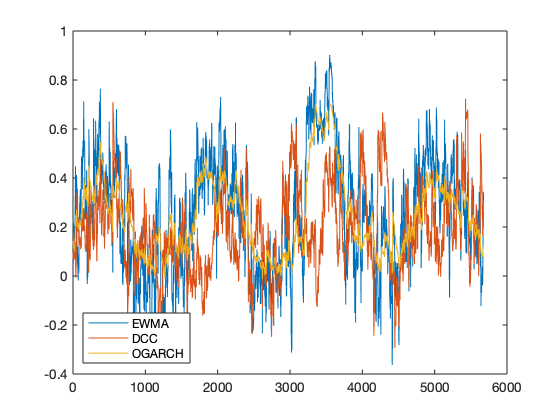Chapter 4: Risk Measures¶

• 4.1/4.2: Expected Shortfall (ES) estimation under normality assumption
In :
% ES in MATLAB
% Listing 4.2
% Last updated August 2016
%
%

p = [0.5,0.1,0.05,0.025,0.01,0.001];
VaR = -norminv(p)
ES = normpdf(norminv(p))./p
VaR =

0    1.2816    1.6449    1.9600    2.3263    3.0902

ES =

0.7979    1.7550    2.0627    2.3378    2.6652    3.3671

Chapter 5: Implementing Risk Forecasts¶

• 5.3/5.4: Univariate HS Value at Risk (VaR)
• 5.5/5.6: Multivariate HS VaR
• 5.7/5.8: Univariate ES VaR
• 5.9/5.10: Normal VaR
• 5.11/5.12: Portfolio Normal VaR
• 5.13/5.14: Student-t VaR (unavailable as of June 2018)
• 5.15/5.16: Normal ES VaR
• 5.17/5.18: Direct Integration Normal ES VaR
• 5.19/5.20: MA Normal VaR
• 5.21/5.22: EWMA VaR
• 5.23/5.24: Two-asset EWMA VaR
• 5.25/5.26: GARCH(1,1) VaR
In :
% Listing 5.2
% Last updated July 2020
%
%

p1 = stocks(:,1);             % consider first two stocks
p2 = stocks(:,2);

y1=diff(log(p1));             % convert prices to returns
y2=diff(log(p2));
y=[y1 y2];
T=length(y1);
value = 1000;                 % portfolio value
p = 0.01;                     % probability
In :
% Univariate HS VaR in MATLAB
% Listing 5.4
% Last updated July 2020
%
%

ys = sort(y1);   % sort returns
op = ceil(T*p);  % p percent smallest, rounded up to meet VaR probability requirement
VaR1 = -ys(op)*value
VaR1 =

17.4982

In :
% Multivariate HS VaR in MATLAB
% Listing 5.6
% Last updated 2011
%
%

w = [0.3; 0.7];    % vector of portfolio weights
yp = y*w;          % portfolio returns
yps = sort(yp);
VaR2 = -yps(op)*value
VaR2 =

18.7263

In :
% Univariate ES in MATLAB
% Listing 5.8
% Last updated 2011
%
%

ES1 = -mean(ys(1:op))*value
ES1 =

22.5634

In :
% Normal VaR in MATLAB
% Listing 5.10
% Last updated 2011
%
%

sigma = std(y1); % estimate volatility
VaR3 = -sigma * norminv(p) * value
VaR3 =

14.9496

In :
% Portfolio normal VaR in MATLAB
% Listing 5.12
% Last updated 2011
%
%

sigma = sqrt(w' * cov(y) * w); % portfolio volatility
VaR4 = - sigma * norminv(p) *  value
VaR4 =

17.0411

In :
% Student-t VaR in MATLAB
% Listing 5.14
% Last updated 2011
%
%

scy1=y1*100;          % scale the returns
res=mle(scy1,'distribution','tlocationscale');
sigma1 = res(2)/100;  % rescale the volatility
nu = res(3);
VaR5 = - sigma1 * tinv(p,nu) * value
VaR5 =

17.1234

In :
% Normal ES in MATLAB
% Listing 5.16
% Last updated June 2018
%
%

sigma = std(y1);
ES2=sigma*normpdf(norminv(p))/p * value
ES2 =

17.1272

In :
% Direct integration ES in MATLAB
% Listing 5.18
% Last updated 2011
%
%

VaR = -norminv(p);
ES =

17.1266

In :
% MA normal VaR in MATLAB
% Listing 5.20
% Last updated June 2018
%
%

WE=20;
for t=T-5:T
t1=t-WE+1;
window=y1(t1:t);  % estimation window
sigma=std(window);
VaR6 = -sigma * norminv(p) * value
end
VaR6 =

16.0505

VaR6 =

16.1491

VaR6 =

18.8543

VaR6 =

18.8821

VaR6 =

16.2305

VaR6 =

16.1698

In :
% EWMA VaR in MATLAB
% Listing 5.22
% Last updated 2011
%
%

lambda = 0.94;
s11 = var(y1(1:30)); % initial variance
for t = 2:T
s11 = lambda * s11  + (1-lambda) * y1(t-1)^2;
end
VaR7 = -norminv(p) * sqrt(s11) * value
VaR7 =

16.7534

In :
% Two-asset EWMA VaR in MATLAB
% Listing 5.24
% Last updated 2011
%
%

s = cov(y);               % initial covariance
for t = 2:T
s = lambda * s +  (1-lambda) * y(t-1,:)' * y(t-1,:);
end
sigma = sqrt(w' * s * w); % portfolio vol
VaR8 = - sigma * norminv(p) * value
VaR8 =

20.5036

In :
% GARCH in MATLAB
% Listing 5.26
% Last updated August 2016
%
%

[parameters,ll,ht]=tarch(y1,1,0,1);
omega = parameters(1)
alpha = parameters(2)
beta = parameters(3)
sigma2 = omega + alpha*y1(end)^2 + beta*ht(end) % calc sigma2 for t+1
VaR9 = -sqrt(sigma2) * norminv(p) * value
____________________________________________________________
Diagnostic Information

Number of variables: 3

Functions
Objective:                            tarch_likelihood
Hessian:                              finite-differencing (or Quasi-Newton)

Algorithm selected
quasi-newton

____________________________________________________________
End diagnostic information
First-order
Iteration  Func-count       f(x)        Step-size       optimality
0           4         -20893.3                          31.2
1          12         -20895.9     0.00481538           15.9
2          16           -20897              1             10
3          20         -20898.6              1           7.49
4          28         -20900.8             10             14
5          32         -20902.8              1           14.4
6          36         -20903.6              1           2.15
7          40         -20903.6              1           1.49
8          44         -20903.6              1          0.456
9          48         -20903.6              1         0.0371
10          52         -20903.6              1         0.0037
11          56         -20903.6              1        0.00152
12         100         -20903.6        2.05571       0.000881

Local minimum possible.

fminunc stopped because it cannot decrease the objective function
along the current search direction.

omega =

9.6212e-07

alpha =

0.0603

beta =

0.9167

sigma2 =

5.2688e-05

VaR9 =

16.8862

Chapter 6: Analytical Value-at-Risk for Options and Bonds¶

• 6.1/6.2: Black-Scholes function definition
• 6.3/6.4: Black-Scholes option price calculation example
In :
% Black-Scholes function in MATLAB
% Listing 6.2
% Last updated 2011
%
%

%% To run this code block in Jupyter notebook:
%% delete all lines above the line with file bs.m, then run

%%file bs.m
function  res = bs(K,P,r,sigma,T)
d1 = (log(P./K)+(r+(sigma^2)/2)*T)./(sigma*sqrt(T));
d2 = d1 - sigma*sqrt(T);
res.Call = P.*normcdf(d1,0,1)-K.*exp(-r*T).*normcdf(d2,0,1);
res.Put = K.*exp(-r*T).*normcdf(-d2,0,1)-P.*normcdf(-d1,0,1);
res.Delta.Call = normcdf(d1,0,1);
res.Delta.Put = res.Delta.Call -1;
res.Gamma = normpdf(d1,0,1)./(P*sigma*sqrt(T));
end
Created file '/Users/alvaroaguirre/SRC/Dropbox/Alvaro/Listings-2020/jupyter/bs.m'.
In :
% Black-Scholes in MATLAB
% Listing 6.4
% Last updated 2011
%
%

f=bs(90,100,0.05,0.2,0.5)
Error using bs
Too many input arguments.

Chapter 7: Simulation Methods for VaR for Options and Bonds¶

• 7.1/7.2: Plotting normal distribution transformation
• 7.3/7.4: Random number generation from Uniform(0,1), Normal(0,1)
• 7.5/7.6: Bond pricing using yield curve
• 7.7/7.8: Yield curve simulations
• 7.9/7.10: Bond price simulations
• 7.11/7.12: Black-Scholes analytical pricing of call
• 7.13/7.14: Black-Scholes Monte Carlo simulation pricing of call
• 7.15/7.16: Option density plots
• 7.17/7.18: VaR simulation of portfolio with only underlying
• 7.19/7.20: VaR simulation of portfolio with only call
• 7.21/7.22: VaR simulation of portfolio with call, put and underlying
• 7.23/7.24: Simulated two-asset returns
• 7.25/7.26: Two-asset portfolio VaR
• 7.27/7.28: Two-asset portfolio VaR with a call
In :
% Transformation in MATLAB
% Listing 7.2
% Last updated July 2020
%
%

x=-3:0.1:3;
plot(x,normcdf(x))
title("CDF of Normal Distribution")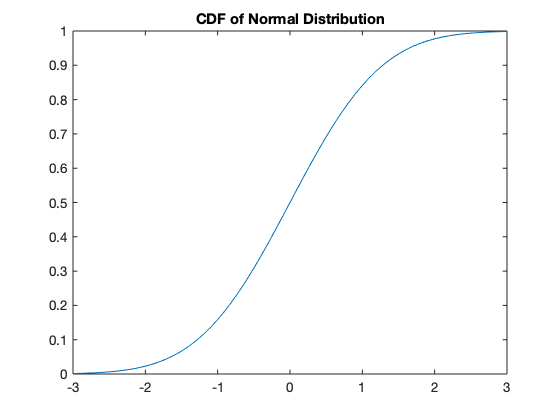In :
% Various RNs in MATLAB
% Listing 7.4
% Last updated August 2016
%
%

rng default; % set seed

S=10;

rand(S,1)
randn(S,1)
trnd(4,S,1)
ans =

0.8147
0.9058
0.1270
0.9134
0.6324
0.0975
0.2785
0.5469
0.9575
0.9649

ans =

-1.3499
3.0349
0.7254
-0.0631
0.7147
-0.2050
-0.1241
1.4897
1.4090
1.4172

ans =

1.2458
-1.8803
0.4892
2.4722
0.5898
1.0088
0.8056
-0.2653
0.2206
-0.8372

In :
% Price bond in MATLAB
% Listing 7.6
% Last updated July 2020
%
%

yield = [5.00 5.69 6.09 6.38 6.61...
6.79 6.94 7.07 7.19 7.30]; % yield curve
T = length(yield);
r=0.07;                             % initial yield rate
Par=10;                             % par value
coupon=r*Par;                       % coupon payments
cc=zeros(1,T)+coupon;               % vector of cash flows
cc(T)=cc(T)+Par;                    % add par to cash flows
P=sum(cc./((1+yield./100).^(1:T)))  % calculate price
P =

9.9132

In :
% Simulate yields in MATLAB
% Listing 7.8
% Last updated July 2020
%
%

randn('state',123);         % set the seed
sigma = 1.5;                % daily yield volatility
S = 8;                      % number of simulations
r = randn(1,S)*sigma;       % generate random numbers

ysim=nan(T,S);
for i=1:S
ysim(:,i)=yield+r(i);
end
ysim=repmat(yield',1,S)+repmat(r,T,1);
plot(ysim)
title("Simulated yield curves")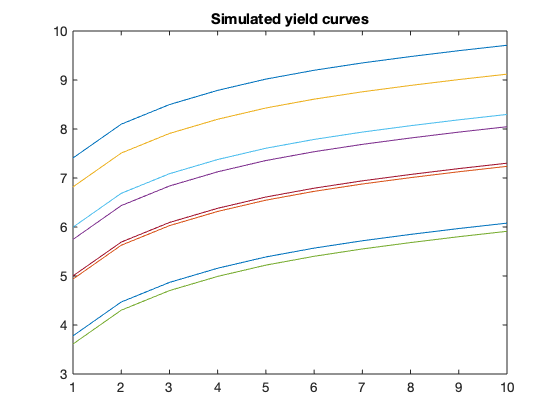In :
% Simulate bond prices in MATLAB
% Listing 7.10
% Last updated July 2020
%
%

SP = nan(S,1);
for s = 1:S                                        % S simulations
SP(s) = sum(cc./(1+ysim(:,s)'./100).^((1:T)));
end
SP = SP-(mean(SP) - P);                            % correct for mean
bar(SP)

S = 50000;
rng("default")
r = randn(S,1) * sigma;
ysim = nan(T,S);
for i = 1:S
ysim(:,i) = yield' + r(i);
end

SP = nan(S,1);
for i = 1:S
SP(i) = sum(cc./(1+ysim(:,i)'./100).^((1:T)));
end

SP = SP  - (mean(SP)-P);

histfit(SP)
title("Histogram of simulated bond prices with fitted normal")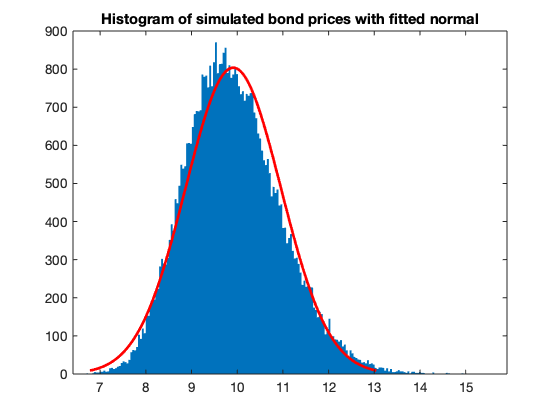In :
% Black-Scholes valuation in MATLAB
% Listing 7.12
% Last updated July 2020
%
%

P0 = 50;                      % initial spot price
sigma = 0.2;                  % annual volatility
r = 0.05;                     % annual interest
T = 0.5;                      % time to expiration
X = 40;                       % strike price
f = bs(X,P0,r,sigma,T)        % analytical call price

%% This calculation uses the Black-Scholes pricing function (Listing 6.1/6.2)
f =

struct with fields:

Call: 11.0873
Put: 0.0997
Delta: [1x1 struct]
Gamma: 0.0107

In :
% Black-Scholes simulation in MATLAB
% Listing 7.14
% Last updated July 2020
%
%

randn('state',0);            % set seed
S = 1e6;                     % number of simulations
ysim = randn(S,1)*sigma*sqrt(T)-0.5*T*sigma^2; % sim returns, lognorm corrected
F = P0*exp(r*T)*exp(ysim);   % sim future prices
SP = F-X;                    % payoff
SP(find(SP < 0)) = 0;        % set negative outcomes to zero
fsim = SP * exp(-r*T) ;      % discount
mean(fsim)                   % simulated price
ans =

11.0996

In :
% Option density plots in MATLAB
% Listing 7.16
% Last updated July 2020
%
%

subplot(1,2,1)
histfit(F);
title("Simulated prices");
xline(X, 'LineWidth', 1, 'label', 'Strike');

subplot(1,2,2)
hist(fsim,100);
title("Option price density");
xline(mean(fsim), 'LineWidth', 1, 'label', 'Call');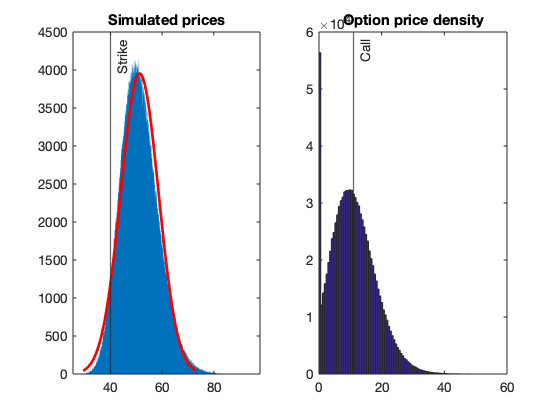In :
% Simulate VaR in MATLAB
% Listing 7.18
% Last updated 2011
%
%

randn('state',0);   % set seed
S = 1e7;            % number of simulations
s2 = 0.01^2;        % daily variance
p = 0.01;           % probability
r = 0.05;           % annual riskfree rate
P = 100;            % price today
ysim = randn(S,1)*sqrt(s2)+r/365-0.5*s2; % sim returns
Psim = P*exp(ysim);  % sim future prices
q = sort(Psim-P);  % simulated P/L
VaR1 = -q(S*p)
VaR1 =

2.2906

In :
% Simulate option VaR in MATLAB
% Listing 7.20
% Last updated 2011
%
%

T = 0.25;                           % time to expiration
X = 100;                            % strike price
sigma = sqrt(s2*250);               % annual volatility
f = bs(X,P,r,sigma,T);              % analytical call price
fsim=bs(X,Psim,r,sigma,T-(1/365));  % sim option prices
q = sort(fsim.Call-f.Call);         % simulated P/L
VaR2 = -q(p*S)
VaR2 =

1.2150

In :
% Example 7.3 in MATLAB
% Listing 7.22
% Last updated 2011
%
%

X1 = 100;
X2 = 110;
f1 = bs(X1,P,r,sigma,T);
f2 = bs(X2,P,r,sigma,T);
f1sim=bs(X1,Psim,r,sigma,T-(1/365));
f2sim=bs(X2,Psim,r,sigma,T-(1/365));
q = sort(f1sim.Call+f2sim.Put+Psim-f1.Call-f2.Put-P);
VaR3 = -q(p*S)
VaR3 =

1.4951

In :
% Simulated two-asset returns in MATLAB
% Listing 7.24
% Last updated 2011
%
%

randn('state',12)                 % set seed
mu = [r/365 r/365]';              % return mean
Sigma=[0.01 0.0005; 0.0005 0.02]; % covariance matrix
y = mvnrnd(mu,Sigma,S);           % simulated returns
In :
% Two-asset VaR in MATLAB
% Listing 7.26
% Last updated 2011
%
%

K = 2;
P = [100 50]';                        % prices
x = [1 1]';                           % number of assets
Port = P'*x;                          % portfolio at t
Psim = repmat(P,1,S)' .*exp(y);       % simulated prices
PortSim=Psim * x;                     % simulated portfolio value
q = sort(PortSim-Port);               % simulated P/L
VaR4 = -q(S*p)
VaR4 =

25.9700

In :
% A two-asset case in MATLAB with an option
% Listing 7.28
% Last updated 2011
%
%

f = bs(P(2),P(2),r,sigma,T);
fsim=bs(P(2),Psim(:,2),r,sigma,T-(1/365));
q = sort(fsim.Call+Psim(:,1)-f.Call-P(1));
VaR5 = -q(p*S)
VaR5 =

20.8125

Chapter 8: Backtesting and Stress Testing¶

• 8.3/8.4: Setting up backtest
• 8.5/8.6: Running backtest for EWMA/MA/HS/GARCH VaR
• 8.7/8.8: Backtesting analysis for EWMA/MA/HS/GARCH VaR
• 8.9/8.10: Bernoulli coverage test
• 8.11/8.12: Independence test
• 8.13/8.14: Running Bernoulli/Independence test on backtests
• 8.15/8.16: Running backtest for EWMA/HS ES
• 8.17/8.18: Backtesting analysis for EWMA/HS ES
In :
% Listing 8.2
% Last updated August 2016
%
%

y=diff(log(price)); % get returns
In :
% Set backtest up in MATLAB
% Listing 8.4
% Last updated July 2020
%
%

T = length(y);  % number of obs for return y
WE = 1000;      % estimation window length
p = 0.01;       % probability
l1 = ceil(WE*p) ;     % HS observation
value = 1;      % portfolio value
VaR = NaN(T,4); % matrix for forecasts
%% EWMA setup
lambda = 0.94;
s11 = var(y);
for t = 2:WE
s11=lambda*s11+(1-lambda)*y(t-1)^2;
end
In [ ]:
% Running backtest in MATLAB
% Listing 8.6
% Last updated June 2018
%
%

for t = WE+1:T
t1 = t-WE;          % start of the data window
t2 = t-1;           % end of data window
window = y(t1:t2) ; % data for estimation
s11 = lambda*s11  + (1-lambda)*y(t-1)^2;
VaR(t,1) = -norminv(p) * sqrt(s11)  *value; % EWMA
VaR(t,2) = -std(window)*norminv(p)*value; % MA
ys = sort(window);
VaR(t,3) = -ys(l1)*value; % HS
[par,ll,ht]=tarch(window,1,0,1);
h=par(1)+par(2)*window(end)^2+par(3)*ht(end);
VaR(t,4) = -norminv(p)*sqrt(h)*value; % GARCH(1,1)
end
In :
% Backtesting analysis in MATLAB
% Listing 8.8
% Last updated July 2020
%
%

names = ["EWMA", "MA", "HS", "GARCH"];
for i=1:4
VR = length(find(y(WE+1:T)<-VaR(WE+1:T,i)))/(p*(T-WE));
s = std(VaR(WE+1:T,i));
disp([names(i), "Violation Ratio:", VR, "Volatility:", s])
end
plot([y(WE+1:T) VaR(WE+1:T,:)])
"EWMA"    "Violation Ratio:"    "2.0744"    "Volatility:"    "0.012114"

"MA"    "Violation Ratio:"    "1.8606"    "Volatility:"    "0.0066223"

"HS"    "Violation Ratio:"    "1.2404"    "Volatility:"    "0.012292"

"GARCH"    "Violation Ratio:"    "1.6895"    "Volatility:"    "0.011511"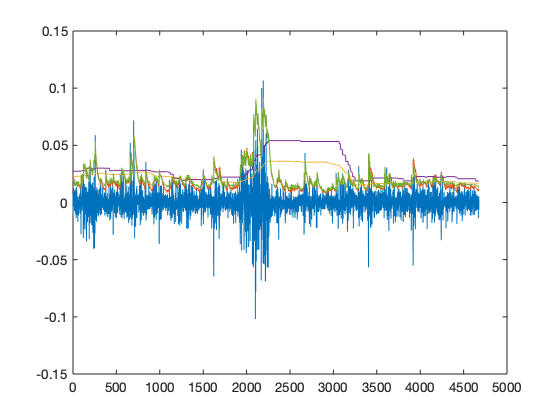In :
% Bernoulli coverage test in MATLAB
% Listing 8.10
% Last updated July 2020
%
%

%% To run this code block in Jupyter notebook:
%% delete all lines above the line with bern_test.m, then run

%%file bern_test.m

function res=bern_test(p,v)
lv = length(v);
sv = sum(v);
al = log(p)*sv + log(1-p)*(lv-sv);
bl = log(sv/lv)*sv + log(1-sv/lv)*(lv-sv)
res=-2*(al-bl);
end
Created file '/Users/alvaroaguirre/SRC/Dropbox/Alvaro/Listings-2020/jupyter/bern_test.m'.
In :
% Independence test in MATLAB
% Listing 8.12
% Last updated July 2020
%
%

%% To run this code block in Jupyter notebook:
%% delete all lines above the line with ind_test.m, then run

%%file ind_test.m

function res=ind_test(V)
T=length(V);
J=zeros(T,4);
for i = 2:T
J(i,1)=V(i-1)==0 & V(i)==0;
J(i,2)=V(i-1)==0 & V(i)==1;
J(i,3)=V(i-1)==1 & V(i)==0;
J(i,4)=V(i-1)==1 & V(i)==1;
end
V_00=sum(J(:,1));
V_01=sum(J(:,2));
V_10=sum(J(:,3));
V_11=sum(J(:,4));
p_00=V_00/(V_00+V_01);
p_01=V_01/(V_00+V_01);
p_10=V_10/(V_10+V_11);
p_11=V_11/(V_10+V_11);
hat_p=(V_01+V_11)/(V_00+V_01+V_10+V_11);

al = log(1-hat_p)*(V_00+V_10) + log(hat_p)*(V_01+V_11);
bl = log(p_00)*V_00 + log(p_01)*V_01 + log(p_10)*V_10 + log(p_11)*V_11;
res= -2*(al-bl);
end
Created file '/Users/alvaroaguirre/SRC/Dropbox/Alvaro/Listings-2020/jupyter/ind_test.m'.
In :
% Backtesting S&P 500 in MATLAB
% Listing 8.14
% Last updated July 2020
%
%

names = ["EWMA", "MA", "HS", "GARCH"];
ya=y(WE+1:T);
VaRa=VaR(WE+1:T,:);
for i=1:4
q=find(y(WE+1:T)<-VaR(WE+1:T,i));
v=VaRa*0;
v(q,i)=1;
ber=bern_test(p,v(:,i));
in=ind_test(v(:,i));
disp([names(i), "Bernoulli Statistic:", ber, "P-value:", 1-chi2cdf(ber,1),...
"Independence Statistic:", in, "P-value:", 1-chi2cdf(in,1)])
end
bl =

-471.9091

Columns 1 through 5

"EWMA"    "Bernoulli Statis..."    "41.6257"    "P-value:"    "1.1053e-10"

Columns 6 through 9

"Independence Sta..."    "0.44111"    "P-value:"    "0.50659"

bl =

-432.8188

Columns 1 through 5

"MA"    "Bernoulli Statis..."    "27.9039"    "P-value:"    "1.2749e-07"

Columns 6 through 9

"Independence Sta..."    "17.4418"    "P-value:"    "2.9624e-05"

bl =

-312.2446

Columns 1 through 5

"HS"    "Bernoulli Statis..."    "2.5354"    "P-value:"    "0.11132"

Columns 6 through 9

"Independence Sta..."    "11.4848"    "P-value:"    "0.00070167"

bl =

-400.7082

Columns 1 through 5

"GARCH"    "Bernoulli Statis..."    "18.6034"    "P-value:"    "1.6094e-05"

Columns 6 through 9

"Independence Sta..."    "0.29841"    "P-value:"    "0.58488"

In :
% Backtest ES in MATLAB
% Listing 8.16
% Last updated 2011
%
%

VaR = NaN(T,2);  % VaR forecasts for 2 models
ES = NaN(T,2);   % ES forecasts for 2 models
for t = WE+1:T
t1 = t-WE;
t2 = t-1;
window = y(t1:t2) ;

s11 = lambda * s11  + (1-lambda) * y(t-1)^2;
VaR(t,1) = -norminv(p) * sqrt(s11)  *value; % EWMA
ES(t,1) = sqrt(s11) * normpdf(norminv(p)) / p;

ys = sort(window);
VaR(t,2) = -ys(l1) * value;          % HS
ES(t,2) = -mean(ys(1:l1)) * value;
end
In :
% ES in MATLAB
% Listing 8.18
% Last updated July 2020
%
%

names = ["EWMA", "HS"];
VaRa = VaR(WE+1:T,:);
ESa = ES(WE+1:T,:);
for i = 1:2
q = find(ya <= -VaRa(:,i));
nES = mean(ya(q) ./ -ESa(q,i));
disp([names(i), nES])
end
"EWMA"    "1.2235"

"HS"    "1.0537"

Chapter 9: Extreme Value Theory¶

• 9.1/9.2: Calculation of tail index from returns
In :
% Hill estimator in MATLAB
% Listing 9.2
% Last updated 2011
%
%

ysort = sort(y);                            % sort the returns
CT = 100;                                   % set the threshold
iota = 1/mean(log(ysort(1:CT)/ysort(CT+1))) % get the tail index

% END
iota =

2.6297